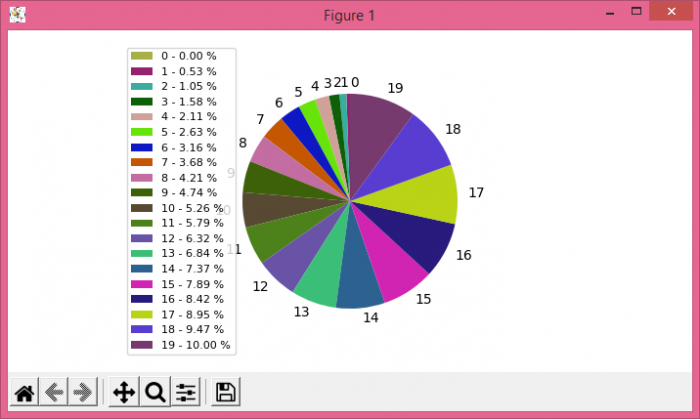# How to avoid overlapping of labels & autopct in a Matplotlib pie chart?

To avoid overlapping of labels and autopct in a matplotlib pie chart, we can follow label as a legend, using legend() method.

## Steps

• Initialize a variable n=20 to get a number of sections in a pie chart.

• Create slices and activities using numpy.

• Create random colors using hexadecimal alphabets, in the range of 20.

• Use pie() method to plot a pie chart with slices, colors, and slices data points as a label.

• Make a list of labels (those are overlapped using autopct).

• Use legend() method to avoid overlapping of labels and autopct.

• To display the figure, use show() method.

## Example

import random
import numpy as np
from matplotlib import pyplot as plt
plt.rcParams["figure.figsize"] = [7.00, 3.50]
plt.rcParams["figure.autolayout"] = True
n = 20
slices = np.arange(n)
activities = np.arange(n)
colors = ["#" + ''.join([random.choice('0123456789ABCDEF') for j in range(6)])
for i in range(n)]

patches, texts = plt.pie(slices,
colors=colors,
startangle=90,
labels=slices)

labels = ['{0} - {1:1.2f} %'.format(i, j) for i, j in zip(activities,
100.*slices/slices.sum())]
plt.legend(patches, labels, loc='center left', bbox_to_anchor=(-0.35, .5), fontsize=8)
plt.show()

## Output